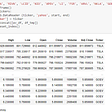# Analyzing 2021 Billionaires using python | Data Science

The dataset I will be using in this project is downloaded from Kaggle. The link to the CSV file is given below

https://www.kaggle.com/roysouravcu/forbes-billionaires-of-2021

We are going to make the most out of this analysis. Let’s Start!

To start we need to import some basic modules such as pandas, NumPy, matplotlib, and seaborn.

`import pandas as pdimport numpy as npimport matplotlib.pyplot as pltimport seaborn as sns`

Now we will load the dataset into a variable named `df`

`df = pd.read_csv("Billionaire.csv")df.head()`

We should get the following output

In the ‘NetWorth’ column we can see that the variables are entered as \$177 B. We need to remove ‘\$’ and ‘B’ and convert them into float for easy reading of numeric figures.

`df['NetWorth'] = df['NetWorth'].str.strip('B') #Removing Bdf['NetWorth'] = df['NetWorth'].str.strip('\$') #Removing \$df['NetWorth'] = df["NetWorth"].astype(float) #Converting to floatdf['NetWorth'] #display variable in output`

We need to remove the rows having missing/null values. For that first let's check which columns have missing values.

`print(df.isnull().sum())`

From this, we can see that the ‘Age’ column has 79 missing values let’s remove that with the following code.

`df = df.dropna()`

Let’s try plotting graph for top 15 Billionaires

For that, we store the Top 15 Billionaire in the new variable ‘df2’ and plot a bar graph where ‘x-axis = Name’ and ‘y-axis = Networth’.

`#Storing top 15 Billionaire in new variabledf2 = df.sort_values(by = ["NetWorth"], ascending=False).head(15)#plotting graphplt.figure(figsize=(20, 25))ax = sns.barplot(x=df2['Name'],y=df2['NetWorth'],data=df2)ax.set_xticklabels(ax.get_xticklabels(), rotation=40, ha="right")plt.show()`

let’s plot a graph for the age of billionaires

`plt.style.use('fivethirtyeight')plt.figure(figsize=(20, 10))ax = sns.displot(df['Age'])plt.ylabel('No of Billionaire')plt.show()`

From this graph we can say that most number of billionaires are at the range of age 50–70.

Now Let’s look at the major Industries(eg. Technology, Fintech, Pharma, Automobile, etc) from where these billionaires emerge.

`i = df['Industry'].value_counts().head(7)index = i.indexcountries = i.values#colorscustom_colors = ["darkorange", "darkslategrey", 'forestgreen', "cornflowerblue", "aqua", "orchid", "lavender"]#plotting graphplt.figure(figsize=(5, 5))plt.pie(countries, labels=index, colors=custom_colors)central_circle = plt.Circle((0, 0), 0.5, color='white')fig = plt.gcf()fig.gca().add_artist(central_circle)plt.rc('font', size=12)plt.title("Top 7Industries with Most Number of Billionaires", fontsize=20)plt.show()`

Similarly, we can look a the Countries having the most number of billionaires.

`i = df['Country'].value_counts().head(7)index = i.indexcountries = i.values#colorscustom_colors = ["darkorange", "darkslategrey", 'forestgreen', "cornflowerblue", "aqua", "orchid", "lavender"]#plotting graphplt.figure(figsize=(5, 5))plt.pie(countries, labels=index, colors=custom_colors)central_circle = plt.Circle((0, 0), 0.5, color='white')fig = plt.gcf()fig.gca().add_artist(central_circle)plt.rc('font', size=12)plt.title("Top 7 Countries with Most Number of Billionaires", fontsize=20)plt.show()`

These were only a few ways to analyze the 2021 Billionaires.

# Over \$1 trillion is needed by 2030 to put the world on track to reach net-zero by 2050

By net-zero, we mean zero carbon emission and other greenhouse gases that are harmful to our planet.

From this project, I found a very interesting number that is \$102.68 Billion. This number was computed by summing up 0.8% Networth of every Billionaire on this list.

`df['0.8% of Networth'] = df['NetWorth']*0.008Total = df['0.8% of Networth'].sum()Total = round(Total,2)print(f'\${Total} B')#Output = \$102.68 B`

So if the Billionaires from around the world together invest 0.8% of their net worth for over 10 years then we can reach a target of \$1 trillion.

I’m not saying that only billionaires are responsible for investing in R&D(research and development) for clean energy, but they can and are helping in a big way✌️.

--

--

--

## More from Jigyanshu Singh

Practicing data science | Implemented effective trading strategies in stock market

Love podcasts or audiobooks? Learn on the go with our new app.

## 7 UNKNOWN APPLICATIONS OF DATA SCIENCE## L-systems: draw a stochastic plant (II)## How I Deployed a Sentiment Analyser API with spaCy, Flask and Heroku## This gave me such great comfort, especially in the darkest days of the Spring when the COVID was…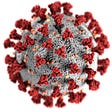## Inductive Link Prediction in Knowledge Graphs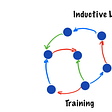## Data Scientists’ baptisms of fire## forwardship## Jigyanshu Singh

Practicing data science | Implemented effective trading strategies in stock market

## Backtesting A Call Spread In Python Using Data In Yahoo Finance## Stock Analysis using Python (TSA).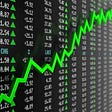## Raindrop Charts in Python in 5 steps.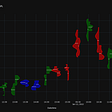## EV Stock Daily Cumulative Returns using Python Pandas